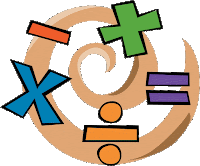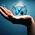## WHY DO WE TAKE ALL THAT MATH ANYWAY?As a student, I asked where on earth I'd use all that math they taught us. As a Grade Six teacher I was asked the same question by MY students. I used to say in cooking, is sewing, if you become a builder, an engineer, or any other profession using math. I still had trouble coming up with examples.

Now I know.

#### THE USE OF MATH permeates our daily lives.

You just never know when math will come in handy, even if you have a calculator.

### FRACTIONS:

When I am baking, I often reduce recipes by on half or one third. Fractions and how to add, subtract, multiply and divide them have served me well. My smaller size cakes and muffins come out just fine.

### GEOMETRY

• When I was an appraiser, measuring houses and calculating their area, I used basic geometry to make my task easier. I divided odd shaped houses into rectangles, triangles and parallelograms to figure out the areas. I used a calculator for the math, but needed to know what math to use.
• Did you know that to find the area of a regular bay window (one that has a floor in it), multiply the longest side (width) by the depth.? Carpenters use shapes and math and measuring to create buildings, furniture and decks.
• When crocheting hats, pi helps. The circumference of a circle is the diameter times Pi.
•• So if the flat crown of the hat is six inches in diameter (the point at which you stop enlarging the circle) then the circumference will be about nineteen inches (6 x 3.142 = 18.8 inches) and will fit a small adult head.
• Adjust your basic crown and you can adjust your hat size. If you want a hat to fit a 14 inch head, you'd make the base @4.5 inches. (4/3.142=4.45 inches)
• Once you know what to do, you can use the calculator for the hard part. But I estimate and do the math in my head. (6" diameter by roughly 3 gives me an  18+ inch size) so I don't have to search for a device.

### ALGEBRA

When appraising high rise buildings and motels and strip malls, I needed to use formula. Thank goodness I learned the proper order in which to deal with brackets and numbers. Things like Cap Rates or future value of the dollar would have been tricky without it. (Of course, now there are computers to do the math, but still, good to have a back up to check things.)

BASIC MATH FUNCTIONS

• When calculating value of several items when I know the price of one,  I use the basic formula.
If 2 = 10,
then 10 = ( 10x10) divided by 2
or 50.

• I estimate my total grocery bill by rounding the price of each item to the nearest dollar and adding the numbers in my head. Back in the day, when budgeting was critical to my financial management and I only had so much money in my pocket, it proved a valuable skill.
• Now that we don't have pennies (here in Canada), rounding is a handy skill  Remember: if the pennies are 1 or 2 - round down. If the pennies are 3 or 4-  round up.  (So \$1.02 counts as a \$1; \$1.04 counts as \$1.05)

My other uses include but are not limited to:
• Figuring out prices of multiples or singles at the grocery store
• Calculating how many words a day I need to write to achieve 60,000 words in 30 days, or how many hours a 900 km trip will take.
• Figuring out the ratio of dry ingredients to liquid to alter or create new recipes.

## Is there anyone out there who NEVER uses numbers and math?

1.2.1.